# JP2004320985A - Inverter controller for driving motor and air conditioner - Google Patents

## Info

Publication number
JP2004320985A
JP2004320985A JP2004074860A JP2004074860A JP2004320985A JP 2004320985 A JP2004320985 A JP 2004320985A JP 2004074860 A JP2004074860 A JP 2004074860A JP 2004074860 A JP2004074860 A JP 2004074860A JP 2004320985 A JP2004320985 A JP 2004320985A
Authority
JP
Japan
Prior art keywords
motor
motor current
value
inverter
means
Prior art date
Legal status (The legal status is an assumption and is not a legal conclusion. Google has not performed a legal analysis and makes no representation as to the accuracy of the status listed.)
Granted
Application number
JP2004074860A
Other languages
Japanese (ja)
Other versions
JP3955287B2 (en
Inventor
Mitsuo Kawachi
Hideo Matsushiro
Toshihiro Sugimoto

Original Assignee
Matsushita Electric Ind Co Ltd

Priority date (The priority date is an assumption and is not a legal conclusion. Google has not performed a legal analysis and makes no representation as to the accuracy of the date listed.)
Filing date
Publication date
Priority to JP2003100008 priority Critical
Application filed by Matsushita Electric Ind Co Ltd, 松下電器産業株式会社 filed Critical Matsushita Electric Ind Co Ltd
Priority to JP2004074860A priority patent/JP3955287B2/en
Publication of JP2004320985A publication Critical patent/JP2004320985A/en
Application granted granted Critical
Publication of JP3955287B2 publication Critical patent/JP3955287B2/en
Application status is Expired - Fee Related legal-status Critical
Anticipated expiration legal-status Critical

• 239000003570 air Substances 0 title 1
• 230000002829 reduced Effects 0 abstract 3
• 239000003990 capacitor Substances 0 abstract 1

## Images

•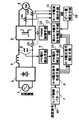•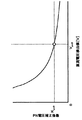•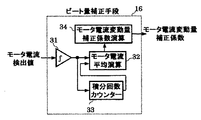•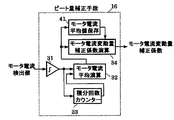•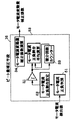•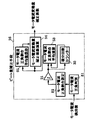•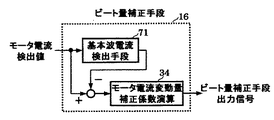•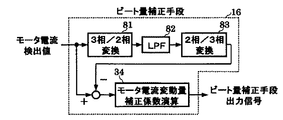•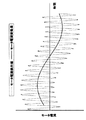•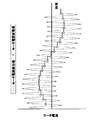•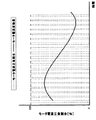•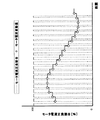•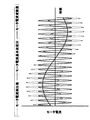•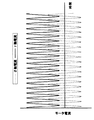•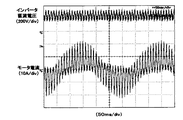•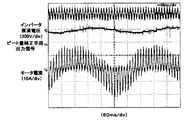•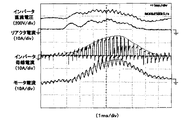••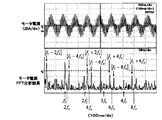••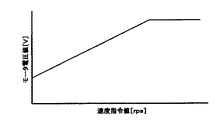•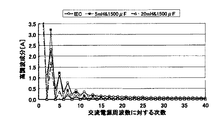•## Classifications

• HELECTRICITY
• H02GENERATION; CONVERSION OR DISTRIBUTION OF ELECTRIC POWER
• H02PCONTROL OR REGULATION OF ELECTRIC MOTORS, ELECTRIC GENERATORS OR DYNAMO-ELECTRIC CONVERTERS; CONTROLLING TRANSFORMERS, REACTORS OR CHOKE COILS
• H02P27/00Arrangements or methods for the control of AC motors characterised by the kind of supply voltage
• H02P27/04Arrangements or methods for the control of AC motors characterised by the kind of supply voltage using variable-frequency supply voltage, e.g. inverter or converter supply voltage
• H02P27/045Arrangements or methods for the control of AC motors characterised by the kind of supply voltage using variable-frequency supply voltage, e.g. inverter or converter supply voltage whereby the speed is regulated by measuring the motor speed and comparing it with a given physical value

## Abstract

PROBLEM TO BE SOLVED: To provide a motor drive inverter control device which is further reduced in size, weight and cost by reducing the amount of change in motor current.
SOLUTION: The use of a small-capacity reactor and a small-capacitance capacitor makes it possible to realize a small, lightweight and low-cost inverter control device for driving a motor. In this case, it is possible to maintain the driving of the motor by making the voltage applied to the motor substantially constant by the PN voltage correction means, and to further reduce the loss by suppressing the fluctuation amount of the motor current by the beat amount correction means. And further reduce the size, weight, and cost of the motor drive inverter control device by reducing the current capacity of the elements.
[Selection diagram] Fig. 1

## Description

The present invention relates to a motor drive inverter control device using a small capacity reactor and a small capacity capacitor, and further relates to an air conditioner using a motor drive inverter control device as the inverter device.

As a general induction motor driving inverter control device used in a general-purpose inverter or the like, a V / F control type induction motor driving inverter control device as shown in FIG. 20 is well known (for example, see Non-Patent Documents). 1).

20, the main circuit includes a DC power supply 203, an inverter 3 and an induction motor 4. The DC power supply 203 includes an AC power supply 1, a rectifier circuit 2, and a DC voltage source of the inverter 3. For this purpose, a smoothing capacitor 202 for storing electric energy and a power factor improving reactor 201 of the AC power supply 1 are constituted.

On the other hand, in the control circuit, a V / F control pattern 7 for determining a motor voltage value to be applied to the induction motor 4 based on an externally given speed command W * of the induction motor 4, and a V / F control pattern 7 Motor voltage generating means 8 for generating a motor voltage command value for the induction motor 4 based on the motor voltage value to be generated, and a PWM signal for the inverter 3 based on the motor voltage command value generated from the motor voltage generating means 8. It comprises a PWM control means 12.

An example of a general V / F control pattern 7 is shown in FIG. As shown in FIG. 21, the motor voltage value applied to the induction motor 4 is uniquely determined with respect to the speed command W * . Generally, the speed command W * and the value of the motor voltage value are stored as a table value in a memory of an arithmetic device such as a microcomputer, and the speed command W * other than the table value is linearly interpolated from the table value. The motor voltage value is derived.

Here, when the AC power supply 1 is 220 V (AC power supply frequency 50 Hz), the input of the inverter 3 is 1.5 kW, and the smoothing capacitor 202 is 1500 μF, the harmonics of the AC power supply current when the power factor improving reactor 201 is 5 mH and 20 mH. FIG. 22 shows the relationship between the wave component and the order with respect to the AC power supply frequency. FIG. 22 shows the results together with the IEC (International Electrotechnical Commission) standard. When the power factor improving reactor 201 is 5 mH, the third harmonic component is much larger than that of the IEC standard. In the case of, it can be seen that the IEC standard is satisfied in the harmonic components up to the 40th order.

Therefore, it is necessary to take measures such as further increasing the inductance value of the power factor improving reactor 201 in order to meet the IEC standard even under a high load, and the inverter device becomes larger, the weight increases, and the cost increases. Was inconvenient.

Therefore, as a DC power supply that suppresses an increase in the inductance value of the power factor improving reactor 201 and achieves a reduction in power supply harmonic components and a high power factor, for example, a DC power supply as shown in FIG. 23 has been proposed. (For example, Patent Document 1).

In FIG. 23, the AC power supply voltage of the AC power supply 1 is applied to an AC input terminal of a full-wave rectifier circuit in which diodes D1 to D4 are bridge-connected, and the output is charged to the intermediate capacitor C via the reactor Lin. The charge of the intermediate capacitor C is discharged to the smoothing capacitor CD to supply a DC voltage to the load resistor RL. In this case, the transistor Q1 is connected to a positive / negative DC current path connecting the load side of the reactor Lin and the intermediate capacitor C, and the transistor Q1 is driven by the base drive circuit G1.

Further, it further includes pulse generation circuits I1 and I2 for applying a pulse voltage to the base drive circuit G1, and a dummy resistor Rdm, wherein each of the pulse generation circuits I1 and I2 detects a zero-cross point of the AC power supply voltage. And a pulse current circuit that supplies a pulse current to the dummy resistor Rdm until the instantaneous value of the AC power supply voltage becomes equal to the voltage across the intermediate capacitor C from the detection of the zero crossing point.

Here, the pulse generation circuit I1 generates a pulse voltage in the first half of a half cycle of the AC power supply voltage, and the pulse generation I2 generates a pulse voltage in the second half of the half cycle of the AC power supply voltage.

When the transistor Q1 is turned on to force a current to flow through the reactor Lin, a backflow prevention diode D5 is connected so that the charge of the intermediate capacitor C is not discharged through the transistor Q1, and the intermediate capacitor C A diode D6 for preventing backflow and a reactor Ldc for enhancing a smoothing effect are connected in series to a path for discharging the electric charge of the above to the smoothing capacitor CD.

With the above configuration, the transistor Q1 is turned on in a part or all of the phase section in which the instantaneous value of the AC power supply voltage does not exceed the voltage between both ends of the intermediate capacitor C. It is possible to achieve a reduction in components and a high power factor.

Inverter Drive Handbook, pages 661 to 711, edited by the Inverter Drive Handbook Editing Committee, 1995 first edition, published by Nikkan Kogyo Shimbun JP-A-9-266677

However, in the above-mentioned conventional configuration, the smoothing capacitor CD having a large capacity and the reactor Lin (the simulation result at 1500 μF and 6.2 mH is described in Patent Document 1) remain as before, and furthermore the intermediate By including the capacitor C, the transistor Q1, the base drive circuit G1, the pulse generation circuits I1 and I2, the dummy resistor Rdm, the backflow prevention diodes D5 and D6, and the reactor Ldc for enhancing the smoothing effect, the device can be increased in size and the number of parts can be reduced. There is a problem that the cost is increased due to the increase in the cost.

The present invention solves such a conventional problem, and provides a motor drive inverter control device that is further reduced in size, weight, and cost by reducing the amount of change in motor current. Aim.

In order to solve the above problems, the present invention is a motor drive inverter control device including a rectifier circuit that receives an AC power supply and an inverter that converts DC power to AC power, wherein the rectifier circuit includes a diode bridge and a diode. The bridge is composed of a predetermined small-capacity reactor connected to the AC input side or DC output side of the bridge, and a predetermined small-capacity capacitor for absorbing the regenerative energy of the motor is provided between the DC buses of the inverter. A motor voltage command generating means for generating a motor voltage command value of the motor based on the motor speed command value given from the motor; a PN voltage detecting means for detecting an inverter DC voltage value; and a preset inverter DC voltage reference. PN voltage correction means for deriving a ratio between the value and a DC voltage detection value of the inverter obtained from the PN voltage detection means; The motor voltage command value is corrected by multiplying the motor voltage command value obtained from the motor voltage command creation means by the PN voltage correction coefficient which is the output value of the PN voltage correction means, and the motor voltage command correction value of the motor is obtained. Motor voltage command correction means for generating the motor current detection means for detecting the motor current of the motor, and the motor current fluctuation value derived from the motor current detection value obtained from the motor current detection means, Beat amount correction means for deriving an antiphase component, and performing voltage correction of the motor voltage command correction value by multiplying a motor voltage command correction value obtained from the motor voltage command correction means and an output value of the beat amount correction means, And a second motor voltage command correcting means for generating a voltage command value to be applied to the motor.

With the above configuration, a small-sized, light-weight, and low-cost inverter control device for motor drive can be realized by using a small-capacity reactor and a small-capacity capacitor. In this case, it is possible to maintain the driving of the motor by making the voltage applied to the motor substantially constant by the PN voltage correction means, and further to suppress the loss by suppressing the fluctuation amount of the motor current by the beat amount correction means. It is possible to further reduce the size, weight, and cost of the motor drive inverter control device by reducing the current and the current capacity of the elements.

The beat amount correction means derives the motor current fluctuation amount by averaging the motor current detection values.

With the above configuration, the motor current fluctuation amount can be derived by a simple calculation, so that it is possible to prevent a large increase in the calculation amount and the memory in the calculation means such as the microcomputer, and to configure the calculation means with the same cost. Is possible.

The beat amount correcting means derives an average value of the motor current detection value for each cycle of the operation frequency of the inverter, and during a period in which the average value of the motor current detection value is derived, determines at least one cycle before the motor current detection value. The average value of the values is defined as the motor current fluctuation amount, and the motor current fluctuation amount is newly updated when the period during which the average value of the motor current detection value is derived ends.

With the above configuration, the average value of the motor current detection value is derived for each cycle of the operating frequency of the inverter, so that the amount of calculation and memory in the calculation means such as a microcomputer can be reduced, and the cost of the calculation means can be reduced. Is possible.

The beat amount correcting means derives a positive / negative ratio of the motor current detection value, and derives a motor current fluctuation amount by averaging the positive / negative ratio.

According to the above configuration, the average calculation of the motor current detection value is performed as it is, but the amount of calculation and memory in the calculation means such as a microcomputer can be reduced, and the cost of the calculation means can be reduced.

Further, the beat amount correction means derives an average value of the positive / negative ratio for each cycle of the operating frequency of the inverter, and in a period in which the average value of the positive / negative ratio is derived, averages the positive / negative ratio at least one cycle before The value is defined as the motor current variation, and the motor current variation is newly updated when the period during which the average value of the positive and negative ratios is derived ends.

With the above configuration, since the average value of the positive / negative ratio is derived for each cycle of the inverter operating frequency, a microcomputer or the like is further required than when the average value of the motor current detection value is derived for each cycle of the inverter operating frequency. It is possible to reduce the amount of calculation and memory in the calculation means, and to reduce the cost of the calculation means.

The beat amount correcting means derives a motor current fluctuation amount by a first-order lag calculation of the motor current detection value.

According to the above configuration, the amount of calculation and the memory in the calculation means such as a microcomputer can be reduced, while the average calculation of the motor current detection value is performed, and the cost of the calculation means can be reduced.

Also, the beat amount correcting means suppresses the motor current fluctuation amount only when the operating frequency of the inverter is higher than the cutoff frequency in the first-order lag calculation.

With the above configuration, an unstable phenomenon such as hunting of the motor current of the motor can be avoided.

Further, the beat amount correcting means includes a delay time compensating means for compensating for a time delay caused by the primary delay calculation.

With the above-described configuration, the delay time associated with the first-order delay calculation can be compensated, so that the effect of suppressing the motor current variation can be improved.

Further, the beat amount correcting means includes a fundamental wave current detecting means for detecting a fundamental wave component of the motor current from the detected motor current value, and the motor current fluctuation is determined by a difference between the motor current detected value and an output value of the fundamental wave current detecting means. Deriving the quantity.

According to the above-described configuration, the amount of calculation and memory in the calculation means such as a microcomputer can be reduced as compared with the first-order delay calculation of the motor current detection value, and the cost of the calculation means can be reduced.

In addition, the fundamental wave current detection means derives a motor current fundamental wave component by performing a first-order lag operation on a three-phase / two-phase conversion of the motor current detection value and further performing a two-phase / three-phase conversion. .

According to the above configuration, since the motor current detection value is converted from three-phase AC to two-phase DC and then the first-order lag calculation is performed, the motor current fluctuation is derived without time delay in real time, thereby suppressing the motor current fluctuation. The effect can be improved.

Further, the motor current fluctuation amount is suppressed only when the motor current fluctuation amount is larger than a preset value of the motor current fluctuation amount.

According to the above configuration, the motor current fluctuation can be effectively suppressed by suppressing the motor current fluctuation only when the motor current fluctuation is larger than a preset value of the motor current fluctuation. Furthermore, the amount of calculation and memory in the calculation means such as a microcomputer can be reduced, and the cost of the calculation means can be reduced.

Further, the motor current fluctuation amount is suppressed only when the operation frequency of the inverter is higher than a preset inverter operation frequency set value.

According to the above configuration, the motor current variation can be effectively suppressed by suppressing the motor current variation only when the operating frequency of the inverter is higher than a preset inverter operating frequency set value. It is possible to reduce the amount of calculation and memory in the calculation means such as a microcomputer, and it is possible to reduce the cost of the calculation means.

In a transient state in which the motor accelerates or decelerates, the motor current fluctuation amount is not suppressed.

With the above configuration, it is possible to avoid an unstable phenomenon such as hunting of the motor current of the motor by not suppressing a motor current fluctuation amount in a transient state where the motor accelerates or decelerates. It is possible to reduce the amount of calculation and memory in the calculating means, and it is possible to reduce the cost of the calculating means.

Further, the motor current detecting means includes a current detector for detecting a current flowing in the bus current of the inverter, and detects a shunt resistance provided in advance on the DC bus of the inverter for overcurrent protection of the inverter. A motor current is detected in synchronization with the carrier frequency from the output value of the current detector.

With the above configuration, it is possible to minimize the cost required for the motor current detection unit as compared with the case where a current sensor is used.

As is clear from the above, the present invention includes a rectifier circuit having an AC power supply as an input, an inverter for converting DC power to AC power, and a motor, the rectifier circuit includes a diode bridge, and an AC input side or DC of the diode bridge. It is composed of a predetermined small-capacity reactor connected to the output side, and a predetermined small-capacity capacitor for absorbing the regenerative energy of the motor is provided between the DC bus of the inverter. A motor voltage command generating means for generating a motor voltage command value of the motor based on the value; a PN voltage detecting means for detecting a DC voltage value of the inverter; and a preset inverter DC voltage reference value and PN voltage detecting means. PN voltage correction means for deriving the ratio between the obtained inverter DC voltage value and motor voltage command generation means. Motor voltage command correction means for performing a voltage correction of the motor voltage command value by multiplying a motor voltage command value to be multiplied by a PN voltage correction coefficient which is an output value of the PN voltage correction means, thereby creating a motor voltage command correction value for the motor. And a motor current detecting means for detecting a motor current of the motor; and a beat amount for deriving an amount of fluctuation of the motor current from a motor current detection value obtained from the motor current detecting means, and deriving an antiphase component of the motor current fluctuation amount. The correction means and the motor voltage command correction value obtained from the motor voltage command correction means are multiplied by the output value of the beat amount correction means to perform the voltage correction of the motor voltage command correction value, and the applied voltage command value to the motor is corrected. And a second motor voltage command correcting means to be created. According to this configuration, a small capacity reactor and a small capacity capacitor are used. This makes it possible to realize a compact, lightweight, and low-cost inverter controller for driving a motor. Even when the DC voltage of the inverter fluctuates greatly and driving of the motor becomes difficult or impossible, the PN voltage correction means applies the voltage to the motor. The motor drive can be maintained by making the applied voltage almost constant, and furthermore, the amount of fluctuation of the motor current is suppressed by the beat amount correction means, thereby reducing the loss and the motor drive inverter control by reducing the element current capacity. There is an effect that the size, weight and cost of the device can be further reduced.

Further, according to the present invention, the beat amount correcting means derives the motor current fluctuation amount by averaging the motor current detection value. According to this configuration, the motor current fluctuation amount can be derived by a simple calculation. Therefore, it is possible to prevent a large increase in the amount of calculation and the memory in the calculation means such as a microcomputer, and it is possible to achieve the effect that the calculation means can be configured with the same cost.

Also, in the present invention, the beat amount correction means derives an average value of the motor current detection value for each cycle of the operating frequency of the inverter, and at least one cycle before the average value of the motor current detection value is derived. The average value of the motor current detection values is used as the motor current fluctuation amount, and the motor current fluctuation amount is newly updated when the period in which the average value of the motor current detection values is derived ends. According to this configuration, Since the average value of the motor current detection value is derived for each cycle of the operating frequency of the inverter, it is possible to reduce the amount of calculation and memory in the calculation means such as a microcomputer, and to reduce the cost of the calculation means. It works.

Further, according to the present invention, the beat amount correcting means derives a positive / negative ratio of the motor current detection value, and derives a motor current fluctuation amount by averaging the positive / negative ratio. Compared to averaging the values as they are, it is possible to reduce the amount of calculation and the memory in the calculation means such as a microcomputer, and to reduce the cost of the calculation means.

Also, in the present invention, the beat amount correction means derives an average value of the positive / negative ratio for each cycle of the operating frequency of the inverter, and in a period in which the average value of the positive / negative ratio is derived, at least one cycle before the positive / negative value. The average value of the ratio of the motor current fluctuation is defined as the motor current fluctuation amount, and the motor current fluctuation amount is updated anew when the period of deriving the average value of the positive and negative ratios ends. The average value of the positive / negative ratio is derived for each cycle of the inverter. This has the effect of reducing the cost of the calculation means.

Further, according to the present invention, the beat amount correcting means derives the motor current fluctuation amount by a first-order lag calculation of the motor current detection value. In addition, it is possible to reduce the amount of calculation and the memory in the calculation means such as a microcomputer, and to reduce the cost of the calculation means.

Further, according to the present invention, the beat amount correcting means suppresses the motor current fluctuation amount only when the operating frequency of the inverter is higher than the cutoff frequency in the first-order lag calculation. This has an effect that an unstable phenomenon such as hunting can be avoided.

Further, according to the present invention, the beat amount correcting means includes a delay time compensating means for compensating for a time delay accompanying the first-order delay calculation. Therefore, the effect of suppressing the motor current fluctuation can be improved.

Further, in the present invention, the beat amount correcting means includes a fundamental wave current detecting means for detecting a fundamental wave component of the motor current from the motor current detected value, and a difference between the motor current detected value and an output value of the fundamental wave current detecting means. According to this configuration, the first-order lag calculation of the motor current detection value is performed, but the amount of calculation and the memory in the calculation means such as a microcomputer can be reduced. This has the effect of enabling cost reduction.

Further, in the present invention, the fundamental wave current detecting means performs a first-order lag operation on a three-phase / two-phase conversion of the motor current detection value, and further derives a motor current fundamental wave component by performing a two-phase / 3-phase conversion. According to this configuration, since the motor current detection value is converted from three-phase AC to two-phase DC and then the first-order lag operation is performed, the motor current fluctuation is derived in real time without time delay. This has the effect that the effect of suppressing the amount can be improved.

Further, the present invention suppresses the motor current fluctuation amount only when the motor current fluctuation amount is larger than a preset value of the motor current fluctuation amount. According to this configuration, the motor current fluctuation amount is set in advance. The motor current variation can be effectively suppressed by suppressing the motor current variation only when the motor current variation is greater than the set value of the set motor current variation. The memory can be reduced, and the cost of the calculation means can be reduced.

Further, the present invention suppresses the motor current fluctuation amount only when the operating frequency of the inverter is higher than a preset inverter operating frequency set value. According to this configuration, the operating frequency of the inverter is set in advance. By suppressing the motor current fluctuation only when it is larger than the inverter operation frequency set value, the motor current fluctuation can be effectively suppressed. Thus, there is an effect that the cost of the calculation means can be reduced.

Further, the present invention does not suppress the motor current fluctuation amount in a transient state in which the motor accelerates or decelerates. According to this configuration, the motor current in the transient state in which the motor accelerates or decelerates By not suppressing the fluctuation amount, an unstable phenomenon such as hunting of the motor current of the motor can be avoided, and furthermore, the calculation amount and memory in the calculation means such as a microcomputer can be reduced, and the cost of the calculation means can be reduced. This has the effect that it is possible.

Further, the motor current detecting means includes a current detector for detecting a current flowing in the bus current of the inverter, and detects a shunt resistance provided in advance on the DC bus of the inverter for overcurrent protection of the inverter. The motor current is detected in synchronization with the carrier frequency from the output value of the current detector. According to this configuration, the cost for the motor current detection means is reduced as compared with the case where the current sensor is used. There is an effect that it can be minimized.

Hereinafter, embodiments of the present invention will be described with reference to the drawings. In the embodiments described below, an inverter control device for driving an induction motor is described. However, the present invention is not limited to the inverter control device for driving an induction motor, and can be applied to all inverter control devices for driving a motor. It is.

(Embodiment 1)
FIG. 1 shows a system configuration diagram of an inverter control device for driving an induction motor according to a first embodiment of the present invention. In FIG. 1, the main circuit includes an AC power supply 1, a diode bridge 2 for converting AC power to DC power, a small capacity reactor 5, a small capacity capacitor 6, an inverter 3 for converting DC power to AC power, and an inverter. An induction motor 4 is driven by the AC power converted by the motor 3.

On the other hand, in the control circuit, a V / F control pattern 7 for determining a motor voltage value to be applied to the induction motor 4 based on a speed command W * given from the outside, and a motor voltage determined from the V / F control pattern 7 Motor voltage generating means 8 for generating a motor voltage command value for the induction motor 4 based on the value, PN voltage detecting means 9 for detecting a DC voltage value of the inverter 3, and a preset DC voltage reference value for the inverter 3. PN voltage correction means 10 for deriving the ratio between the DC voltage detection value of the inverter 3 obtained from the PN voltage detection means 9 and the motor voltage command value obtained from the motor voltage command creation means 8 and the output value of the PN voltage correction means 10 Is multiplied by the PN voltage correction coefficient, and the motor voltage command value is corrected for the motor voltage of the induction motor 4. Motor voltage command correction means 11 for creating a command correction value, motor current detection means 15 for detecting motor current by inverter bus current detector 13 or motor current detectors 14a and 14b, and a motor obtained from motor current detection means 15. Beat amount correction means 16 for detecting the amount of change in motor current from the detected current value and deriving an anti-phase component of the motor current change amount, and motor voltage command correction value and beat amount correction means obtained from motor voltage command correction means 11. A second motor voltage command correction unit 17 for performing voltage correction of the motor voltage command correction value by multiplying the output value of the second motor voltage command value by multiplying the output value of the second motor voltage command correction value by the second motor voltage command correction value; PWM control means 1 for generating a PWM signal for inverter 3 based on an applied voltage command value created from And 2.

Since the V / F control pattern 7 has been described in the above-described conventional technique, the description is omitted here (the general induction motor driving inverter control device in FIG. 20).

Hereinafter, a specific method will be described.

The motor voltage command creating means creates a motor voltage command value v j * (j = u, v, w) by an operation represented by Expression (1) (hereinafter, similarly, j = u, v, w). .

Here, V m is the motor voltage value determined from the V / F control pattern 7, theta 1 is derived by time integrating the speed command W * as expressed by the number (2).

In the case of performing an arithmetic operation by the arithmetic unit such as a microcomputer, a discrete-time system shall take (sampling time and T S such as a microcomputer), and so the current value nT S to those otherwise stated in the calculation It shall be used (e.g., the theta 1 number (1) is calculated as theta 1 [nT S] in the case of deriving the motor voltage command value).

FIG. 2 is a diagram showing a first embodiment of the PN voltage correcting means 10 according to the present invention. The PN voltage correcting means 10 includes a preset DC voltage reference value Vpn0 of the inverter 3 and a PN voltage detecting means. The PN voltage correction coefficient k pn is derived as shown in Expression (3) using the DC voltage detection value v pn of the inverter 3 obtained from 10.

Here, in the present invention, since a small-capacity capacitor is used, the DC voltage detection value vpn may become zero. Therefore, it is necessary to set a minute term δ 0 to prevent zero division.

It should be noted that, in place of the minute term δ 0 of the equation (3), when the DC voltage detection value v pn is equal to or less than zero, the PN voltage correction coefficient k pn is set to the preset maximum value of the PN voltage correction coefficient. It is possible to prevent zero percent discount.

That is, the PN voltage correction coefficient k pn may be derived as in equation (4).

Here, k pn — max is a preset maximum value of the PN voltage correction coefficient.

Note that the PN voltage correction means 10 maintains the drive of the induction motor even when the inverter DC voltage fluctuates greatly, since the PN voltage correction coefficient kpn has at least a preset upper limit value or lower limit value. It is possible to further suppress the fluctuation of the AC power supply current by having a preset upper limit or lower limit, to improve the AC power supply power factor and to suppress the harmonic component of the AC power supply current. Become.

When the DC voltage detection value is larger than the DC voltage reference value, the PN voltage correction means 10 increases the PN voltage correction coefficient k pn in proportion to the DC voltage detection value, so that the inverter DC voltage is greatly reduced. Even if it fluctuates, it is possible to maintain the driving of the induction motor, and when the inverter DC voltage is larger than the DC voltage reference value, the output torque of the induction motor is improved by increasing the PN voltage correction coefficient. Can be achieved.

Further, the motor voltage command correction means 11 derives the motor voltage command correction value v jh * as shown in Expression (5) using the motor voltage command value v j * and the PN voltage correction coefficient k pn .

Here, FIG. 19 shows the result of frequency analysis of the motor current. In the present invention, since a small capacity capacitor is used, the inverter DC voltage greatly pulsates at twice the frequency of the AC power supply frequency f S , and as shown in FIG. 19, the motor current includes the inverter operation frequency f 1 and the power supply frequency f S. The so-called beat phenomenon occurs in which the variation of the frequency component of the absolute value | f 1 ± 2nf S | (where n is a positive integer) of the sum and difference with the even-numbered frequency is superimposed, This leads to an increase in loss or an increase in the size and cost due to an increase in the current capacity of the element.

At this time, the specifications of the AC power supply 1 were 220 V (AC power supply frequency was 50 Hz), the inductance value of the small capacity reactor 5 was 0.5 mH, the capacity of the small capacity capacitor 6 was 10 μF, and the inverter operating frequency was 90 Hz. is there.

Therefore, the fluctuation amount of the motor current is suppressed by the following method.

FIG. 3 is a diagram showing a first embodiment of the beat amount correction means 16 according to the present invention. When the motor current detection value ij is integrated by the integrator 31, the integration number counter 33 outputs the motor current detection value i. The number of integrations N in one cycle of j is stored, and the motor current averaging section 32 divides the output value of the integrator 31 (motor current integration value) by the number of integrations N stored in the integration number counter 33 to obtain a motor. to derive the current average value i j _ I mean.

Here, the motor current detection value i j in FIG. 19, the number for generally as (6) may be the combination of the fundamental wave component i 1j and variation △ i j, of the motor current detection value i j It is understood that the motor current fluctuation amount △ ij can be derived by the average calculation. (It is obvious because averaging the sine wave or cosine wave results in zero.)

Note that the motor current fundamental wave component i 1j can be expressed as in equation (7).

Here, I 1m is the maximum value of the motor current fundamental wave component, and φ is the power factor angle (the phase difference between the motor voltage and the motor current).

Thus, the motor current average value i j _ mean is expressed as Expression (8).

That is, in equation (8), the motor current detection value i j [(n−N + 1) T S ] from the (N−1) th previous motor current detection value i j [nT S ] is integrated, and the integration is performed. The current value i j — mean [nT s ] of the average value of the motor current is derived by dividing the calculated value by the number of integrations N.

Also derived by calculation of the number (9) the phases of the beat amount correction coefficient k bj by deriving the inverse phase component of the motor current average value in the motor current variation amount correction coefficient calculating unit 34 i j _ mean.

Here, I R is the preset motor current reference value, K B is a control gain.

The relation between the motor current detection value i j and the motor current variation amount △ i j in the beat amount correction means 3 in FIG. Motor current fluctuation amount by deriving a motor current average value i j _ mean the operation of the equation (8) with respect to the motor current detection value i j △ i j is found that has been derived.

As described above, since the motor current fluctuation amount can be derived by a simple calculation, it is possible to prevent a large increase in the calculation amount and the memory in the calculation means such as the microcomputer, and it is possible to configure the calculation means with the same cost. It is.

Next, the second motor voltage command correction means 17 uses the motor voltage command correction value v jh * and the beat amount correction coefficient k bj to derive the applied voltage command value v jh2 * as shown in Expression (10).

In the inverter control for driving an induction motor according to the present invention, the resonance frequency is such that the inverter operation frequency is an even multiple of the AC power supply frequency, and a frequency range having a predetermined frequency width before and after the resonance frequency. By preventing the inverter operating frequency from being constantly fixed, the unstable operation of the induction motor can be prevented, and stable driving can be realized.

In the inverter control device for driving an induction motor of the present invention, the combination of the small-capacity reactor and the small-capacity capacitor is determined so that the resonance frequency of the small-capacity reactor and the small-capacity capacitor is larger than 40 times the AC power supply frequency. This makes it possible to suppress the harmonic component of the AC power supply current and clear the IEC standard.

In the inverter control device for driving an induction motor according to the present invention, the capacitance of the small-capacity capacitor is determined such that the maximum value of the DC voltage value that rises when the inverter stops is smaller than the withstand voltage of the element. Circuit destruction can be prevented.

In the inverter control device for driving an induction motor according to the present invention, the carrier frequency of the inverter is determined so as to satisfy a preset AC power factor, thereby utilizing the carrier frequency dependency of the small-capacity reactor. By setting the required minimum carrier frequency, it is possible to suppress inverter loss to the required minimum.

As described above, the inverter control device of the present embodiment corrects each phase voltage command value using the PN voltage correction coefficient, so that a substantially constant motor voltage is applied even if the PN voltage fluctuates. Therefore, a large-capacity capacitor is not required, and a small-capacity capacitor can be used. By using a small-capacity capacitor, the input current is always supplied to the motor, and the power factor of the input current is improved, so that the reactor can be downsized. By using a small-capacity reactor and a small-capacity capacitor, a compact, lightweight, and low-cost inverter controller for driving an induction motor can be realized, and the inverter DC voltage fluctuates greatly, making it difficult or impossible to drive the induction motor. In this case, the inverter is operated so that the voltage applied to the induction motor is substantially constant, and the drive of the induction motor can be maintained. Further, by suppressing the fluctuation amount of the motor current by the beat amount correcting means, it is possible to further reduce the size, weight and cost of the inverter control device for driving the induction motor by reducing the loss and the current capacity of the element. .

It should be noted that the present invention is not limited to the inverter control device for driving an induction motor by V / F control as in the above-described embodiment, and the present invention is also applicable to a known inverter control device for driving a motor by vector control. It is possible.

Note that the present invention is applicable to both cases where a speed sensor such as a pulse generator cannot be used as in a compressor drive motor of an air conditioner and a case where a speed sensor such as a servo drive can be provided. Is applicable.

(Embodiment 2)
FIG. 4 shows a second embodiment of the beat amount correcting means according to the present invention. The same components as those of the beat amount correction means in FIG. 3 are denoted by the same reference numerals, and the description thereof will be omitted because they are duplicated, and only different portions will be described here.

4, the motor current average calculation unit 32 derives the motor current average value i j _ mean every period of the inverter operation frequency f 1, the motor current average value storage unit 41 is derived by the motor current average calculation section 32 motor is intended to store up value before M cycles of the current average value i j _ mean, the motor of at least one period before the period in which derives the motor current average value i j _ mean (1~M cycle before) the current average value i j _ mean a motor current fluctuation amount △ i j, in which period that derives the motor current average value i j _ mean is newly updated motor current fluctuation amount △ i j if End .

Regarding the number of the motor current average value i j _ mean to store in the motor current average value storage unit 41 M (save up to M cycles previous value), the inverter operation frequency f 1 in view of the delay time and responsiveness Or according to load conditions.

The relation between the motor current detection value i j and the motor current variation amount △ i j in the beat amount correction means 4 in FIG. 10. A motor current average value i j _ mean that ○ mark is derived by calculation in FIG. 10, the motor current average value i j _ mean one cycle before the time period that derives the motor current average value i j _ mean motor current fluctuation amount △ and i j, in which period that derives the motor current detection value i j _ mean is newly updated motor current fluctuation amount △ i j if finished.

Here, FIGS. 15 and 16 show the results when the inverter control device for driving an induction motor of the present invention was operated. Figure 15 is a operation result when no inhibition of motor current fluctuation amount △ i j, period 16, which derives the case (motor current average value i j _ mean that the suppression of the motor current fluctuation amount △ i j a 1 is the previous cycle of the motor current average value i j _ mean as the motor current fluctuation amount △ i j) operation result of the, the motor current fluctuation amount △ i j is the motor current in Figure 16 relative to Figure 15 It can be seen that it has been reduced.

At this time, the specifications of the AC power supply were 220 V (AC power supply frequency was 50 Hz), the inductance value of the small capacity reactor was 0.5 mH, the capacity of the small capacity capacitor was 10 μF, and the inverter operating frequency was 96 Hz.

As described above, since the average value of the motor current detection value is derived for each cycle of the operating frequency of the inverter, it is possible to reduce the amount of calculation and memory in the calculation means such as a microcomputer, and to reduce the cost of the calculation means. It is.

(Embodiment 3)
FIG. 5 shows a third embodiment of the beat amount correcting means according to the present invention. In FIG. 5, a motor current positive / negative determining unit 51 for determining whether the motor current detected value ij is positive or negative, and a motor current positive / negative ratio setting for setting a motor current positive / negative ratio ε j based on an output value of the motor current positive / negative determining unit 51. and parts 52 stores the integral number N in one period of the motor current positive and negative percentage epsilon j at integral number counter 33 when integrated by the integrator 31 the motor current negative ratio epsilon j, motor current negative ratio average calculation The section 53 derives the motor current positive / negative ratio average value ε j — mean by dividing the output value of the integrator 31 (the integral value of the motor current positive / negative ratio ε j ) by the integration number N stored in the integration number counter 33. It is.

Here, the motor current positive / negative ratio ε j is set as shown in Expression (11).

Also, the motor current positive / negative ratio average value ε j — mean is expressed as in Expression (12).

That is, in the equation (12), the motor current positive / negative ratio ε j [(n−N + 1) T S ] from (N−1) times before to the current motor current positive / negative ratio ε j [nT S ] is integrated, and the integration is performed. The current value ε j — mean [nT S ] of the average value of the motor current positive / negative ratio is derived by dividing the calculated value by the number of integrations N.

In addition, the motor current variation correction coefficient calculation unit 34 derives a beat amount correction coefficient k bj of each phase by calculating the equation (13) by deriving an opposite phase component of the motor current positive / negative ratio average value ε j — mean. .

Here, K B is a control gain.

The relation between motor current negative ratio epsilon j and the motor current fluctuation amount △ i j in the beat amount correction means in FIG. 5 in FIG. 11. Motor current fluctuation amount by deriving a motor current negative ratio average value epsilon j _ mean the operation of the equation (12) with respect to the motor current negative ratio ε j △ K B is seen that are derived.

As described above, while the average calculation of the motor current detection value is performed as it is, the amount of calculation and the memory in the calculation means such as a microcomputer can be reduced, and the cost of the calculation means can be reduced.

(Embodiment 4)
FIG. 6 shows a fourth embodiment of the beat amount correcting means according to the present invention. The same components as those of the beat amount correcting means in FIG. 5 are denoted by the same reference numerals, and the description thereof will be omitted because they are duplicated, and only different portions will be described here.

6, a motor current positive / negative ratio average calculating unit 53 derives a motor current positive / negative ratio average value ε j _mean for each cycle of the inverter operating frequency f 1 , and a motor current positive / negative ratio average value storage unit 61 outputs a motor current positive / negative ratio. It is intended to store up value before M period of the motor current positive and negative rate average value epsilon j _ mean derived by the average calculation section 53, in the period in which derives the motor current negative ratio average value epsilon j _ mean at least one cycle before the motor current negative ratio average value epsilon j _ mean motor current fluctuation amount △ i j of (1 to M cycles before), the period in which to derive a motor current negative ratio average value epsilon j _ mean the Is completed, the motor current variation △ ij is newly updated.

Regarding the number M of the motor current positive / negative ratio average values ε j — mean stored in the motor current positive / negative ratio average value storage unit 61 (up to a value before M cycles), the inverter is considered in consideration of delay time and responsiveness. set in advance according to the operation frequency f 1 and load conditions.

The relation between motor current negative ratio epsilon j and the motor current fluctuation amount △ i j in the beat amount correction means in FIG. 6 to FIG. In FIG. 12, the circles indicate the motor current positive / negative ratio average value ε j _mean derived by calculation, and during the period in which the motor current positive / negative ratio average value ε j _mean is derived, the motor current positive / negative ratio average one cycle before is derived. The value ε j — mean is defined as the motor current variation △ ij, and the motor current variation △ ij is updated anew when the period of deriving the motor current positive / negative ratio average ε j — mean ends. .

As described above, since the average value of the positive / negative ratio is derived for each cycle of the inverter operating frequency, the calculation of the microcomputer and the like is further performed than when the average value of the motor current detection value is derived for each cycle of the inverter operating frequency. It is possible to reduce the calculation amount and the memory in the means, and it is possible to reduce the cost of the calculation means.

(Embodiment 5)
A specific method according to the fifth embodiment of the beat amount correcting means according to the present invention will be described below.

The motor current fluctuation amount △ ij is derived by performing a first-order lag operation on the motor current detection value ij , and the motor current fluctuation amount 表ij is represented by Expression (14).

Here, K P is a proportional gain, T is a time constant of the first-order lag calculation, and P is a differential operator.

As described above, the amount of calculation and the amount of memory in the calculation means such as a microcomputer can be reduced as compared with the average calculation of the motor current detection value, and the cost of the calculation means can be reduced.

If the operating frequency f 1 of the inverter is smaller than the cut-off frequency f cut-off in the first-order lag calculation, the motor current detection value ij itself is output to the motor current variation △ ij according to equation (14). is, it is not possible to derive a motor current fluctuation amount △ i j, in which the operating frequency f 1 of the inverter is the suppression of the cut-off frequency f is greater than the cut-off only the motor current fluctuation amount △ i j .

Here, there is a relationship as shown in Expression (15) between the cutoff frequency f cut-off and the time constant T.

As described above, it is possible to avoid an unstable phenomenon in which the motor current of the induction motor hunts.

When the value of the time constant T is large, the time delay associated with the first-order lag calculation becomes large, and the motor current fluctuation amount derived by the first-order lag calculation becomes large with respect to the actual motor current fluctuation amount. Since the delay is delayed and the effect of suppressing the motor fluctuation amount is reduced, a delay time compensating means for compensating for a time delay accompanying the first-order delay calculation is provided.

As described above, since the delay time associated with the first-order delay calculation can be compensated, it is possible to improve the effect of suppressing the motor current fluctuation amount.

(Embodiment 6)
FIG. 7 shows a sixth embodiment of the beat amount correcting means according to the present invention. 7, includes a fundamental current detecting means 71 for detecting the motor current fundamental wave component i 1j from the motor current detection value i j, the difference between the output value i 1j of the motor current detection value i j and the fundamental wave current detecting means Is used to derive the motor current variation.

Here, the motor current detection value ij can be substantially a combination of the fundamental wave component i1j and the variation △ ij , as described above in the equation (6) of the first embodiment.

Therefore, by detecting the motor current fundamental wave component i 1j from the motor current detection value i j, the motor current fluctuation amount as in equation (16) by the difference between the motor current detection value i j and the motor current fundamental wave component i 1jij can be derived.

As described above, the amount of calculation and the amount of memory in the calculation means such as a microcomputer can be reduced in comparison with the first-order delay calculation of the motor current detection value, and the cost of the calculation means can be reduced.

FIG. 8 shows a first embodiment of the fundamental wave current detecting means 71 in FIG.

8, the motor current detection value ij is converted from a three-phase alternating current to a two-phase direct current by a three-phase / two-phase converter 81, and the output value of the three-phase / two-phase converter 81 (motor current variation Δi j The two-phase DC current including ripples is passed through the LPF 72 to make only a complete DC component, and the output value (complete DC component) of the LPF 82 is converted from two-phase DC to three-phase AC by the two-phase / 3-phase converter 83. By performing the conversion, the motor current fundamental wave component i 1j is detected.

Here, the three-phase / two-phase converter 81 converts the motor current detection value ij from the three-phase AC to the two-phase DC iγ, iδ by the calculation of Expression (17).

Further, the LPF 82 calculates only the complete DC components iγ 0 and iδ 0 from the two-phase DC iγ and iδ by the calculation of Expression (18).

Here, K LPF is a proportional gain, T LPF is a time constant, and P is a differential operator.

Further, the two-phase / three-phase converter 83 converts the two-phase direct currents iγ 0 and iδ 0 , which are complete DC components, into a three-phase alternating current by the operation of Expression (19).

Further, the motor current variation correction coefficient calculation unit 34 derives the inverse phase component of the motor current variation △ ij derived by Expression (16), and thereby calculates the beat amount correction coefficient k bj of each phase by Expression (20). Is derived by the calculation of

Here, I R is the preset motor current reference value, K B is a control gain.

FIGS. 13 and 14 are explanatory diagrams of the operation of the beat amount correcting means of FIG. FIG. 13 shows the relationship among the detected motor current value i j , the motor current fundamental wave component i 1j , and the motor current fluctuation amount △ ij , and FIG. 14 shows the output value 2 of the three-phase / two-phase converter 81. This shows phase direct currents iγ and iδ.

Here, the two-phase direct currents iγ and iδ in FIG. 14 are obtained by calculating the motor current detection value ij in FIG. 13 by the equation (17), and the two-phase direct currents iγ and iδ are passed through the LPF 82 to complete the direct current. 2-phase DC i? 0 is a component derives i? 0, the two-phase direct current i? 0, to obtain the motor current fundamental wave component i 1j of Figure 13 by calculating a i? 0 by the number (19). Further, the motor current fluctuation amount △ ij can be obtained by the difference calculation of Expression (16).

In addition, regarding the delay time and the responsiveness of the LPF 82, since the calculation of the equation (18) is performed by two-phase DC, the influence of the delay time by the LPF 82 is actually obtained when the motor current fundamental wave component i 1j is derived. Is decreasing.

As described above, since the motor current detection value is converted from the three-phase AC to the two-phase DC and then the first-order lag calculation is performed, the motor current fluctuation is derived in real time without a time delay, thereby suppressing the motor current fluctuation. It is possible to improve.

(Embodiment 7)
A specific method relating to the condition in the suppression of the motor current fluctuation amount of the beat amount correction means according to the present invention will be described below.

When the motor current fluctuation amount △ ij is smaller than a preset motor current fluctuation amount set value △ i R, the effect of the motor current fluctuation amount △ ij is small, so that the motor current fluctuation amount △ ij is suppressed. stop, in which the suppression of the motor current fluctuation amount △ i j only when the motor current fluctuation amount △ i j is greater than the set value △ i R of a preset motor current fluctuation amount.

As described above, the motor current fluctuation can be effectively suppressed by suppressing the motor current fluctuation only when the motor current fluctuation is larger than the preset value of the motor current fluctuation. It is possible to reduce the amount of calculation and memory in the calculation means such as a microcomputer, and it is possible to reduce the cost of the calculation means.

Moreover, in which the suppression of the motor current fluctuation amount △ i j only if the operation frequency f 1 of the inverter is greater than the inverter operation frequency setting value f 1R that is set in advance.

As described above, it is possible to effectively suppress the motor current fluctuation amount by suppressing the motor current fluctuation amount only when the inverter operation frequency is higher than the preset inverter operation frequency setting value. It is possible to reduce the amount of calculation and memory in the calculation means, and to reduce the cost of the calculation means.

Further, in a transient state in which the induction motor accelerates or decelerates, the motor current fluctuation amount △ ij is not suppressed.

As described above, an unstable phenomenon such as a hunting of the motor current of the induction motor can be avoided by not suppressing the motor current fluctuation amount in a transient state in which the induction motor accelerates or decelerates. It is possible to reduce the amount of calculation and memory in the calculating means, and it is possible to reduce the cost of the calculating means.

(Embodiment 8)
A specific method according to the first embodiment of the motor current detecting means according to the present invention will be described below.

The motor current detecting means includes a current detector that detects a current flowing in the bus current of the inverter, and uses a shunt resistor provided in advance in the DC bus of the inverter as a current detector for overcurrent protection of the inverter. Also, the motor current is detected in synchronization with the carrier frequency from the output value of the current detector.

Here, a first operation explanatory diagram of the current detecting means according to the present invention is shown in FIG. In FIG. 17, the inverter bus current is not a continuous value on the time axis (horizontal axis) like the motor current, but a discrete value for each carrier frequency of the inverter (since a positive current flows into the inverter when the inverter is energized). 17), the inverter bus current detector 13 (a shunt resistor provided in advance for overcurrent protection of the inverter) in FIG. 1 synchronizes with the carrier cycle. It is necessary to detect the inverter bus current.

Also, as shown by the broken line in FIG. 17, the peak value of the inverter bus current (the maximum value in the pulse waveform) substantially matches the motor current. Therefore, the motor current is detected by detecting the peak value of the pulse waveform of the inverter bus current. It is possible to detect.

If the current is detected without synchronizing with the carrier frequency, there is a possibility that the current is detected when the inverter is not energized, which makes it difficult to detect the motor current.

The specifications in FIG. 17 are as follows: the AC power supply is 220 V (AC power supply frequency is 50 Hz), the inductance value of the small capacity reactor is 2 mH, the capacity of the small capacity capacitor is 25 μF, the inverter operation frequency is 40 Hz, and the inverter carrier frequency is 3 .3 kHz.

Here, the motor currents for three phases can be continuously detected by using at least two current detectors like the motor current detectors 14a and 15a in FIG. It is only possible to obtain the motor current for one phase represented by the value.

However, as shown in FIG. 18, the motor current fluctuation amount in the motor current of each phase has a time difference (horizontal axis direction) but the same magnitude and the same period. Is derived by the above-described beat amount correction means, and the V-phase and W-phase motor current fluctuations are set in advance in accordance with the inverter operating frequency and the load conditions, so that the delay time is set in advance from the U-phase motor current fluctuations. V-phase and W-phase motor current fluctuations can be obtained.

Further, the applied voltage command value of the induction motor can be derived by the calculation of the above equation (10) using the motor current fluctuation amount of each phase.

FIG. 18 is a diagram for explaining a second operation of the current detecting means according to the present invention. As the specifications at this time, the AC power supply is 220 V (AC power supply frequency is 50 Hz), and the inductance value of the small capacity reactor is 0.1. 5 mH, the capacity of the small capacitor is 10 μF, the inverter operating frequency is 96 Hz, and the inverter carrier frequency is 5 kHz.

With the above configuration, it is possible to minimize the cost required for the motor current detection unit as compared with the case where a current sensor is used.
Although the above embodiment has been described with respect to the induction motor, the present invention can be applied to other motors.

1 is a system configuration diagram of an induction motor driving inverter control device according to a first embodiment of the present invention. FIG. 1 is a diagram showing a first embodiment of a PN voltage correction unit according to the present invention. FIG. 3 is a diagram showing a first embodiment of a beat amount correction unit according to the present invention. FIG. 6 is a diagram showing a second embodiment of the beat amount correcting means according to the present invention. FIG. 9 is a diagram showing a third embodiment of the beat amount correcting means according to the present invention. FIG. 9 is a diagram showing a fourth embodiment of the beat amount correcting means according to the present invention. FIG. 9 is a diagram showing a sixth embodiment of the beat amount correcting means according to the present invention. FIG. 7 is a diagram showing a first embodiment of a fundamental wave current detecting means in the beat amount correcting means of FIG. 7; Operation explanatory diagram of the beat amount correction means of FIG. Operation explanatory diagram of the beat amount correction means of FIG. Operation explanatory diagram of the beat amount correction means of FIG. Operation explanatory diagram of the beat amount correction means of FIG. FIG. 7 is a diagram illustrating a first operation of the beat amount correction unit in FIG. FIG. 7 is a diagram illustrating a second operation of the beat amount correction unit in FIG. FIG. 4 is a diagram showing a first operation result of the inverter control device for driving an induction motor of the present invention. The figure which shows the 2nd operation result of the inverter control apparatus for the induction motor drive of this invention. First operation explanatory diagram of the motor current detecting means according to the present invention FIG. 3 is a diagram illustrating a second operation of the motor current detecting means according to the present invention. Diagram showing frequency analysis result of motor current System configuration diagram of general inverter controller for driving induction motor FIG. 3 is a diagram illustrating an example of a general V / F control pattern. FIG. 20 is a diagram showing a relationship between a harmonic component of an AC power supply current and an order with respect to the AC power supply frequency in the induction motor driving inverter device of FIG. 20. Configuration diagram of conventional DC power supply

Explanation of reference numerals

DESCRIPTION OF SYMBOLS 1 AC power supply 2 Diode bridge 3 Inverter 4 Induction motor 5 Small capacity reactor 6 Small capacity capacitor 7 V / F control pattern 8 Motor voltage command creation means 9 PN voltage detection means 10 PN voltage correction means 11 Motor voltage command correction means 12 PWM control Means 13 Inverter bus current detector 14a, 15b Motor current detector 15 Motor current detection means 16 Beat amount correction means 17 Second motor voltage command correction means 31 Integrator 32 Motor current average calculation unit 33 Integration number counter 34 Motor current fluctuation amount Correction coefficient calculation unit 41 Motor current average value storage unit 51 Motor current positive / negative determination unit 52 Motor current positive / negative ratio setting unit 53 Motor current positive / negative ratio average calculation unit 61 Motor current positive / negative ratio average value storage unit 71 Fundamental wave current detection means 81 3-phase / 2 phase converter 82 LPF
83 2-phase / 3-phase converter

## Claims (20)

1.   In a motor drive inverter control device including a rectifier circuit having an AC power supply as an input and an inverter for converting DC power to AC power, the rectifier circuit is connected to a diode bridge and an AC input side or a DC output side of the diode bridge. A predetermined small-capacity capacitor for absorbing the regenerative energy of the motor is provided between the DC buses of the inverter, and a speed command value of the motor given from the outside is provided between the DC buses of the inverter. Motor voltage command generating means for generating a motor voltage command value for the motor, a PN voltage detecting means for detecting a DC voltage value of the inverter, and a preset DC voltage reference value for the inverter and a PN voltage detection value. PN voltage correction means for deriving a ratio between the DC voltage detection value of the inverter obtained from the means and The motor voltage command value is corrected by multiplying the motor voltage command value obtained from the motor voltage command creation means by a PN voltage correction coefficient which is an output value of the PN voltage correction means, and the motor of the motor is controlled. A motor voltage command correction unit for creating a voltage command correction value, a motor current detection unit for detecting a motor current of the motor, and a motor current variation value derived from the motor current detection value obtained from the motor current detection unit. A beat amount correction means for deriving an antiphase component of the motor current fluctuation amount; and a motor voltage command correction value obtained from the motor voltage command correction means multiplied by an output value of the beat amount correction means to multiply the motor value. A second motor voltage command correction for performing voltage correction of the voltage command correction value and creating a voltage command value to be applied to the motor; Motor drive inverter control apparatus characterized by comprising a stage.
2.   2. The motor drive inverter control device according to claim 1, wherein the beat amount correction means derives the motor current fluctuation amount by averaging the motor current detection value.
3.   The beat amount correction means derives an average value of the motor current detection value for each cycle of the operating frequency of the inverter, and during the period in which the average value of the motor current detection value is derived, detects the motor current detection value at least one cycle before. An average value of the motor current fluctuation amount is used as the motor current fluctuation amount, and the motor current fluctuation amount is newly updated when a period in which the average value of the motor current detection value is derived ends. An inverter control device for driving a motor according to any one of the above.
4.   The motor drive inverter control device according to claim 1, wherein the beat amount correction means derives a positive / negative ratio of the motor current detection value, and derives the motor current fluctuation amount by averaging the positive / negative ratio. .
5.   The beat amount correction means derives an average value of the positive / negative ratio for each cycle of the operation frequency of the inverter, and in a period in which the average value of the positive / negative ratio is derived, at least one cycle before the positive / negative ratio. 5. The motor current fluctuation amount according to claim 1, wherein the motor current fluctuation amount is newly updated when a period during which the average value of the positive / negative ratio is derived ends. 3. The inverter control device for driving a motor according to claim 1.
6.   2. The motor drive inverter control device according to claim 1, wherein the beat amount correction unit derives the motor current fluctuation amount by a first-order lag calculation of a motor current detection value.
7.   7. The motor drive inverter control device according to claim 6, wherein the beat amount correction unit suppresses the motor current fluctuation amount only when the operation frequency of the inverter is higher than a cutoff frequency in a first-order lag calculation.
8.   8. The motor drive inverter control device according to claim 6, wherein the beat amount correcting unit includes a delay time compensating unit that compensates for a time delay caused by a first-order delay calculation. 9.
9.   The beat amount correcting means includes a fundamental wave current detecting means for detecting a fundamental wave component of the motor current from the motor current detected value, and the motor current is calculated based on a difference between the motor current detected value and an output value of the fundamental wave current detecting means. 2. The motor drive inverter control device according to claim 1, wherein the variation amount is derived.
10.   The fundamental wave current detection means calculates a first-order lag of a three-phase / two-phase conversion of the motor current detection value, and further derives the motor current fundamental component by performing a two-phase / three-phase conversion. The motor drive inverter control device according to claim 9, wherein
11.   The motor drive according to any one of claims 1 to 10, wherein the motor current variation is suppressed only when the motor current variation is larger than a preset value of the motor current variation. Inverter control device.
12.   The motor drive inverter control according to any one of claims 1 to 11, wherein the motor current variation is suppressed only when the operation frequency of the inverter is higher than a preset inverter operation frequency set value. apparatus.
13.   The motor drive inverter control device according to any one of claims 1 to 12, wherein the motor current fluctuation amount is not suppressed in a transient state in which the motor accelerates or decelerates.
14.   The motor drive inverter control device according to any one of claims 1 to 13, wherein the motor current detection means includes a current detector for detecting a current flowing to an AC output side of the inverter.
15.   The motor current detecting means includes a current detector that detects a current flowing through a DC bus of an inverter, and detects the motor current from an output value of the current detector. An inverter control device for driving a motor according to any one of the above.
16.   16. The motor drive inverter control device according to claim 14, wherein the current detector is a current sensor.
17.   16. The motor drive inverter control device according to claim 14, wherein the current detector is a shunt resistor.
18.   18. The motor drive inverter control according to claim 15, wherein a shunt resistor provided in advance on a DC bus of the inverter for overcurrent protection of the inverter is also used as the current detector. apparatus.
19.   19. The motor drive inverter control device according to claim 14, wherein the motor current detection means detects the motor current in synchronization with a carrier frequency of an inverter.
20. An air conditioner comprising: a converter device for converting AC power into DC power; and an inverter device for converting the DC power converted by the converter device into AC power having a variable voltage and a variable frequency and supplying the AC power to a compressor drive motor. An air conditioner, wherein the inverter device according to any one of claims 1 to 19 is used as the inverter device.
JP2004074860A 2003-04-03 2004-03-16 Inverter control device for motor drive and air conditioner Expired - Fee Related JP3955287B2 (en)

## Priority Applications (2)

Application Number Priority Date Filing Date Title
JP2003100008 2003-04-03
JP2004074860A JP3955287B2 (en) 2003-04-03 2004-03-16 Inverter control device for motor drive and air conditioner

## Applications Claiming Priority (3)

Application Number Priority Date Filing Date Title
JP2004074860A JP3955287B2 (en) 2003-04-03 2004-03-16 Inverter control device for motor drive and air conditioner
US10/814,190 US6958589B2 (en) 2003-04-03 2004-04-01 Inverter controller for driving motor and air conditioner using inverter controller
CNB200410034265XA CN1319268C (en) 2003-04-03 2004-04-05 Inverter controller for driving motor and air conditioner using the same

## Publications (2)

Publication Number Publication Date
JP2004320985A true JP2004320985A (en) 2004-11-11
JP3955287B2 JP3955287B2 (en) 2007-08-08

# Family

## Family Applications (1)

Application Number Title Priority Date Filing Date
JP2004074860A Expired - Fee Related JP3955287B2 (en) 2003-04-03 2004-03-16 Inverter control device for motor drive and air conditioner

## Country Status (3)

US (1) US6958589B2 (en)
JP (1) JP3955287B2 (en)
CN (1) CN1319268C (en)

## Cited By (16)

* Cited by examiner, † Cited by third party
Publication number Priority date Publication date Assignee Title
JP2005130690A (en) * 2003-10-03 2005-05-19 Matsushita Electric Ind Co Ltd Motor driving inverter control device and air-conditioning apparatus
JP2006340486A (en) * 2005-06-01 2006-12-14 Fuji Electric Fa Components & Systems Co Ltd Control device of motor
JP2007166690A (en) * 2005-12-09 2007-06-28 Hitachi Appliances Inc Motor control device
WO2007116873A1 (en) * 2006-04-03 2007-10-18 Panasonic Corporation Inverter device and air conditioner
WO2007125826A1 (en) * 2006-04-24 2007-11-08 Panasonic Corporation Inverter device and air conditioner
JP2008109768A (en) * 2006-10-25 2008-05-08 Matsushita Electric Ind Co Ltd Motor driving inverter controller and apparatus equipped therewith
JP2009147993A (en) * 2007-12-11 2009-07-02 Mitsubishi Electric Corp Inverter controller, electric motor drive device and air conditioner
JP2009159750A (en) * 2007-12-27 2009-07-16 Panasonic Corp Failure detector for motor
JP2009247099A (en) * 2008-03-31 2009-10-22 Denso Corp Device for driving three-phase alternating-current synchronous motors
WO2010109520A1 (en) * 2009-03-25 2010-09-30 三菱電機株式会社 Device and method for rotating electric machine
WO2012086010A1 (en) * 2010-12-21 2012-06-28 三菱電機株式会社 Heat pump device, heat pump system, and method for controlling three-phase inverter
JP5658812B1 (en) * 2013-11-19 2015-01-28 シャープ株式会社 Motor control device and refrigeration / air-conditioning device
US9543887B2 (en) 2010-10-15 2017-01-10 Mitsubishi Electric Corporation Heat pump device, heat pump system, and method for controlling three-phase inverter
CN106549620A (en) * 2016-10-26 2017-03-29 中冶南方（武汉）自动化有限公司 A kind of Speed Sensorless Induction Motor vector control system low frequency processing method
US9829226B2 (en) 2011-04-28 2017-11-28 Mitsubishi Electric Corporation Heat pump device, heat pump system, and method for controlling inverter
KR101910942B1 (en) 2016-10-13 2018-10-23 오텍캐리어 주식회사 Apparatus and method for sensing reversed phase of input source in air conditioner

## Families Citing this family (28)

* Cited by examiner, † Cited by third party
Publication number Priority date Publication date Assignee Title
KR100840733B1 (en) * 2002-01-05 2008-06-24 엘지전자 주식회사 Method and system for processing packet data in a communications system and receiving unit thereof
US7122988B2 (en) * 2002-09-13 2006-10-17 Tokyo Electron Limited Rotation drive device and rotation drive method
GB2396491B (en) * 2002-12-21 2005-11-30 Dyson Ltd Power conversion apparatus
JP2004289985A (en) * 2003-03-25 2004-10-14 Matsushita Electric Ind Co Ltd Inverter controller for driving motor and air conditioner
JP3955285B2 (en) * 2003-03-27 2007-08-08 松下電器産業株式会社 Inverter control device for motor drive and air conditioner
JP3980005B2 (en) * 2003-03-28 2007-09-19 松下電器産業株式会社 Inverter control device for motor drive and air conditioner
JP3955286B2 (en) * 2003-04-03 2007-08-08 松下電器産業株式会社 Inverter control device for motor drive and air conditioner
GB2410847A (en) * 2004-02-05 2005-08-10 Dyson Ltd Control of motor winding energisation according to rotor angle
JP3942605B2 (en) * 2004-05-17 2007-07-11 東芝テック株式会社 Motor control device and electrical equipment
US20060204383A1 (en) * 2005-03-08 2006-09-14 Hiroyuki Kushida Electric vacuum cleaner
US8021469B2 (en) * 2005-07-14 2011-09-20 Access Business Group International Llc Control methods for an air treatment system
JP4079962B2 (en) * 2005-08-30 2008-04-23 東芝コンシューママーケティング株式会社 Electric vacuum cleaner
JP4856493B2 (en) * 2006-08-07 2012-01-18 本田技研工業株式会社 2-motor simultaneous drive system and its control device
EP2034605B1 (en) * 2006-08-31 2012-03-28 Mitsubishi Electric Corporation Electric motor driving device, and compressor driving device
ES2321675A1 (en) * 2006-11-16 2009-06-09 Ls Industrial Systems Co., Ltd. Inverter device of bridge in h for alternate current motor.
US7495410B2 (en) * 2007-01-30 2009-02-24 Rockwell Automation Technologies, Inc. Systems and methods for improved motor drive power factor control
JP4637148B2 (en) * 2007-08-27 2011-02-23 日立アプライアンス株式会社 Power converter
GB201003456D0 (en) * 2010-03-02 2010-04-14 Trw Ltd Current sensor error compensation
TWI403871B (en) 2010-10-25 2013-08-01 Ind Tech Res Inst Feedback switching device and method for servo motor driving
DE102012215155A1 (en) * 2012-08-27 2014-02-27 Robert Bosch Gmbh Method for regulating the current intensity of the electrical current flowing through an inductive load, and corresponding circuit arrangement
US8994320B2 (en) * 2013-06-28 2015-03-31 Eaton Corporation System and method for controlling regenerating energy in an adjustable speed drive
JP5742980B1 (en) * 2014-02-19 2015-07-01 ダイキン工業株式会社 Power converter control method
US9787246B2 (en) * 2014-03-15 2017-10-10 Mitsubishi Electric Corporation Motor drive control device, compressor, air-sending device, and air-conditioning apparatus
JP6614825B2 (en) * 2015-06-30 2019-12-04 日立ジョンソンコントロールズ空調株式会社 Power conversion device, motor drive device, refrigeration device
JP6538477B2 (en) * 2015-08-18 2019-07-03 日本電産サーボ株式会社 Motor unit
CN105089999A (en) * 2015-08-20 2015-11-25 深圳市建滔科技有限公司 Power conversion efficiency improvement device of inverter compressor
JP2019004616A (en) * 2017-06-15 2019-01-10 三菱重工サーマルシステムズ株式会社 Inverter device, air conditioner, control method for inverter device, and program
JP2019004617A (en) * 2017-06-15 2019-01-10 三菱重工サーマルシステムズ株式会社 Inverter device, air conditioner, control method for inverter device, and program

## Family Cites Families (25)

* Cited by examiner, † Cited by third party
Publication number Priority date Publication date Assignee Title
JP2780263B2 (en) * 1988-02-23 1998-07-30 株式会社明電舎 Vector control method and apparatus for an induction motor
US4992718A (en) * 1989-09-11 1991-02-12 Nihon Patent Electric Co., Ltd. Electric floor cleaner with a soft start function
US5187419A (en) * 1991-05-06 1993-02-16 Allen-Bradley Company, Inc. Electric motor control apparatus and method
US5206757A (en) * 1992-02-13 1993-04-27 Billy Cheng Collapsible field glasses
US6002218A (en) * 1992-11-20 1999-12-14 Fujitsu General Limited Control device for air conditioner
US5457375A (en) * 1994-05-27 1995-10-10 Emerson Electric Co. Sensorless commutation controller for a poly-phase dynamoelectric machine
JPH0835712A (en) * 1994-07-26 1996-02-06 Fujitsu General Ltd Controller for air conditioner
JP3295553B2 (en) * 1994-10-05 2002-06-24 三菱電機株式会社 Variable speed device
US5561595A (en) * 1995-03-24 1996-10-01 Magl Power Inc. Power inverter with input line conditioning
JP3519540B2 (en) 1996-03-28 2004-04-19 東芝キヤリア株式会社 DC power supply and air conditioner
DE69914242T2 (en) * 1998-03-23 2004-11-04 Hitachi, Ltd. Control device for a brushless motor and machine with a brushless motor
JP4154679B2 (en) * 1998-04-18 2008-09-24 株式会社安川電機 Electric motor power failure processing method and control device therefor
US6229278B1 (en) * 1999-09-29 2001-05-08 Rockwell Technologies, Llc Voltage and current limiting method and apparatus for a voltage/frequency drive
US6489692B1 (en) * 1999-12-13 2002-12-03 Capstone Turbine Corporation Method and apparatus for controlling rotation of magnetic rotor
US6414455B1 (en) * 2000-04-03 2002-07-02 Alvin J. Watson System and method for variable drive pump control
JP4465129B2 (en) * 2000-07-14 2010-05-19 パナソニック株式会社 Brushless motor driving apparatus and driving method
JP4357967B2 (en) * 2002-03-22 2009-11-04 パナソニック株式会社 Control device for synchronous reluctance motor
US6768284B2 (en) * 2002-09-30 2004-07-27 Eaton Corporation Method and compensation modulator for dynamically controlling induction machine regenerating energy flow and direct current bus voltage for an adjustable frequency drive system
EP1843463B1 (en) * 2002-12-12 2013-07-03 Panasonic Corporation Motor control apparatus
JP2004289985A (en) * 2003-03-25 2004-10-14 Matsushita Electric Ind Co Ltd Inverter controller for driving motor and air conditioner
JP3955285B2 (en) * 2003-03-27 2007-08-08 松下電器産業株式会社 Inverter control device for motor drive and air conditioner
JP3980005B2 (en) * 2003-03-28 2007-09-19 松下電器産業株式会社 Inverter control device for motor drive and air conditioner
JP3955286B2 (en) * 2003-04-03 2007-08-08 松下電器産業株式会社 Inverter control device for motor drive and air conditioner
JP2004350493A (en) * 2003-04-28 2004-12-09 Matsushita Electric Ind Co Ltd Inverter controller for driving motor and air conditioner using the same
US7078876B2 (en) * 2003-07-07 2006-07-18 Pentadyne Power Corporation Feedforward controller for synchronous reluctance machines

## Cited By (26)

* Cited by examiner, † Cited by third party
Publication number Priority date Publication date Assignee Title
JP4561219B2 (en) * 2003-10-03 2010-10-13 パナソニック株式会社 Inverter control device for motor drive and air conditioner using the same
JP2005130690A (en) * 2003-10-03 2005-05-19 Matsushita Electric Ind Co Ltd Motor driving inverter control device and air-conditioning apparatus
JP2006340486A (en) * 2005-06-01 2006-12-14 Fuji Electric Fa Components & Systems Co Ltd Control device of motor
JP2007166690A (en) * 2005-12-09 2007-06-28 Hitachi Appliances Inc Motor control device
WO2007116873A1 (en) * 2006-04-03 2007-10-18 Panasonic Corporation Inverter device and air conditioner
JP5114387B2 (en) * 2006-04-03 2013-01-09 パナソニック株式会社 Inverter device and air conditioner
WO2007125826A1 (en) * 2006-04-24 2007-11-08 Panasonic Corporation Inverter device and air conditioner
JP5161766B2 (en) * 2006-04-24 2013-03-13 パナソニック株式会社 Inverter device and air conditioner
JP2008109768A (en) * 2006-10-25 2008-05-08 Matsushita Electric Ind Co Ltd Motor driving inverter controller and apparatus equipped therewith
JP2009147993A (en) * 2007-12-11 2009-07-02 Mitsubishi Electric Corp Inverter controller, electric motor drive device and air conditioner
JP2009159750A (en) * 2007-12-27 2009-07-16 Panasonic Corp Failure detector for motor
JP2009247099A (en) * 2008-03-31 2009-10-22 Denso Corp Device for driving three-phase alternating-current synchronous motors
RU2481694C1 (en) * 2009-03-25 2013-05-10 Мицубиси Электрик Корпорейшн Control device and control method of electric rotary machine
WO2010109520A1 (en) * 2009-03-25 2010-09-30 三菱電機株式会社 Device and method for rotating electric machine
US8610388B2 (en) 2009-03-25 2013-12-17 Mitsubishi Electric Corporation Control apparatus and control method for electric rotating machine
US9543887B2 (en) 2010-10-15 2017-01-10 Mitsubishi Electric Corporation Heat pump device, heat pump system, and method for controlling three-phase inverter
CN103270376A (en) * 2010-12-21 2013-08-28 三菱电机株式会社 Heat pump device, heat pump system, and method for controlling three-hase inverter
WO2012086010A1 (en) * 2010-12-21 2012-06-28 三菱電機株式会社 Heat pump device, heat pump system, and method for controlling three-phase inverter
US9618249B2 (en) 2010-12-21 2017-04-11 Mitsubishi Electric Corporation Heat pump device, heat pump system, and method for controlling three-phase inverter
JP5490260B2 (en) * 2010-12-21 2014-05-14 三菱電機株式会社 Heat pump device, heat pump system, and inverter control method
US9829226B2 (en) 2011-04-28 2017-11-28 Mitsubishi Electric Corporation Heat pump device, heat pump system, and method for controlling inverter
WO2015075980A1 (en) * 2013-11-19 2015-05-28 シャープ株式会社 Motor control device and refrigerating/air-conditioning device
JP5658812B1 (en) * 2013-11-19 2015-01-28 シャープ株式会社 Motor control device and refrigeration / air-conditioning device
KR101910942B1 (en) 2016-10-13 2018-10-23 오텍캐리어 주식회사 Apparatus and method for sensing reversed phase of input source in air conditioner
CN106549620A (en) * 2016-10-26 2017-03-29 中冶南方（武汉）自动化有限公司 A kind of Speed Sensorless Induction Motor vector control system low frequency processing method
CN106549620B (en) * 2016-10-26 2019-07-23 中冶南方（武汉）自动化有限公司 A kind of Speed Sensorless Induction Motor vector control system low frequency processing method

## Also Published As

Publication number Publication date
JP3955287B2 (en) 2007-08-08
US6958589B2 (en) 2005-10-25
CN1319268C (en) 2007-05-30
US20040232877A1 (en) 2004-11-25
CN1543057A (en) 2004-11-03

## Similar Documents

Publication Publication Date Title
CN1037650C (en) Controller for power converter
EP1833153B1 (en) Electric engine start system with active rectifier
KR101325650B1 (en) Power converters
US7898197B2 (en) Motor control device
JP4706324B2 (en) Control device for motor drive system
EP1465333A2 (en) Inverter control circuit and air conditioning system using the same
JP5297953B2 (en) Electric motor drive system for electric vehicle
US5184057A (en) Control method and device for ac motor
US7855526B2 (en) Power conversion control device, power conversion control method, and power conversion control program
US6771522B2 (en) Inverter parallel operation system
US7592765B2 (en) Method and system for controlling synchronous motor drive systems
JP3123079B2 (en) Pwm control converter control device
US9257931B2 (en) Power conversion apparatus
AU713034B2 (en) Multilevel power converting apparatus
US20110279071A1 (en) Control device for ac motor
TWI442690B (en) Instantaneous voltage drop compensation circuit, power conversion device, instantaneous voltage drop compensation method and instantaneous voltage drop compensation program
AU2008227057A1 (en) Motor drive using flux adjustment to control power factor
JP4693904B2 (en) Electric motor drive device and compressor drive device
KR100666812B1 (en) Motor control apparatus
CN1319268C (en) Inverter controller for driving motor and air conditioner using the same
US8542502B2 (en) Electrical power conversion apparatus
JP2004289985A (en) Inverter controller for driving motor and air conditioner
CN101647186B (en) Power conversion system
KR960005691B1 (en) Power converter apparatus
JP3955285B2 (en) Inverter control device for motor drive and air conditioner

## Legal Events

Date Code Title Description
A621 Written request for application examination

Free format text: JAPANESE INTERMEDIATE CODE: A621

Effective date: 20050127

A131 Notification of reasons for refusal

Free format text: JAPANESE INTERMEDIATE CODE: A131

Effective date: 20061031

RD03 Notification of appointment of power of attorney

Free format text: JAPANESE INTERMEDIATE CODE: A7423

Effective date: 20061206

A521 Written amendment

Free format text: JAPANESE INTERMEDIATE CODE: A523

Effective date: 20061213

TRDD Decision of grant or rejection written
A01 Written decision to grant a patent or to grant a registration (utility model)

Free format text: JAPANESE INTERMEDIATE CODE: A01

Effective date: 20070403

A61 First payment of annual fees (during grant procedure)

Free format text: JAPANESE INTERMEDIATE CODE: A61

Effective date: 20070501

R150 Certificate of patent or registration of utility model

Free format text: JAPANESE INTERMEDIATE CODE: R150

FPAY Renewal fee payment (event date is renewal date of database)

Free format text: PAYMENT UNTIL: 20110511

Year of fee payment: 4

FPAY Renewal fee payment (event date is renewal date of database)

Free format text: PAYMENT UNTIL: 20120511

Year of fee payment: 5

FPAY Renewal fee payment (event date is renewal date of database)

Free format text: PAYMENT UNTIL: 20130511

Year of fee payment: 6

FPAY Renewal fee payment (event date is renewal date of database)

Free format text: PAYMENT UNTIL: 20130511

Year of fee payment: 6

FPAY Renewal fee payment (event date is renewal date of database)

Free format text: PAYMENT UNTIL: 20140511

Year of fee payment: 7

LAPS Cancellation because of no payment of annual fees